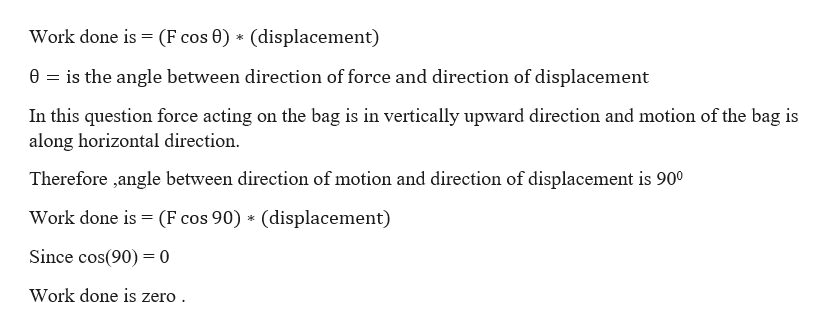You carry a 7.0-kg bag of groceries 1.2 m above the ground at a constant speed accross a 2.7 m room. How much work do you do on the bag in the process

Question

You carry a 7.0-kg bag of groceries 1.2 m above the ground at a constant speed accross a 2.7 m room. How much work do you do on the bag in the process

Step 1

Since the person is holding the bag and moving with constant velocity there will be  only one force  acting on ...help_outlineImage TranscriptioncloseWork done is = (F cos 0) (displacement) 0 is the angle between direction of force and direction of displacement In this question force acting on the bag is in vertically upward direction and motion of the bag is along horizontal direction Therefore ,angle between direction of motion and direction of displacement is 900 Work done is (F cos 90) (displacement) Since cos(90)0 Work done is zero fullscreen

Want to see the full answer?

See Solution

Want to see this answer and more?

Our solutions are written by experts, many with advanced degrees, and available 24/7

See Solution
Tagged in

Work,Power and Energy